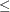### Example 6.18 Migration to OPTMODEL: Production, Inventory, Distribution

The following example shows how to solve Example 6.4 using PROC OPTMODEL. The input data sets are the same as in that example.

The following PROC OPTMODEL code read the data sets, build the linear programming model, solve the model, and output the optimal solution to SAS data sets called `ARC1` and `NODE2`:

```proc optmodel;
set <str> NODES;
num _supdem_ {NODES} init 0;
read data node0 into NODES=[_node_] _supdem_;

set <str,str> ARCS;
num _lo_ {ARCS} init 0;
num _capac_ {ARCS} init .;
num _cost_ {ARCS};
num diagonal {ARCS};
num factory {ARCS};
str key_id {ARCS};
str _name_ {ARCS};
NODES = NODES union (union {<i,j> in ARCS} {i,j});

var Flow {<i,j> in ARCS} >= _lo_[i,j];
for {<i,j> in ARCS: _capac_[i,j] ne .} Flow[i,j].ub = _capac_[i,j];
min obj = sum {<i,j> in ARCS} _cost_[i,j] * Flow[i,j];
con balance {i in NODES}:
sum {<(i),j> in ARCS} Flow[i,j]
- sum {<j,(i)> in ARCS} Flow[j,i] = _supdem_[i];

num infinity = min {r in {}} r;
num excess = sum {i in NODES} _supdem_[i];
if (excess > 0) then do;
/* change equality constraint to le constraint for supply nodes */
for {i in NODES: _supdem_[i] > 0} balance[i].lb = -infinity;
end;
else if (excess < 0) then do;
/* change equality constraint to ge constraint for demand nodes */
for {i in NODES: _supdem_[i] < 0} balance[i].ub = infinity;
end;

solve;

num _supply_ {<i,j> in ARCS} =
(if _supdem_[i] ne 0 then _supdem_[i] else .);
num _demand_ {<i,j> in ARCS} =
(if _supdem_[j] ne 0 then -_supdem_[j] else .);
num _fcost_ {<i,j> in ARCS} = _cost_[i,j] * Flow[i,j].sol;

create data arc1 from [_tail_ _head_]
_cost_ _capac_ _lo_ _name_ _supply_ _demand_ _flow_=Flow _fcost_
_rcost_ =
_status_ = Flow.status diagonal factory key_id mth_made;
create data node2 from [_node_]
_supdem_ = (if _supdem_[_node_] ne 0 then _supdem_[_node_] else .)
_dual_ = balance.dual;
quit;
```

The statements use both single-dimensional (NODES) and multiple-dimensional (ARCS) index sets, which are populated from the corresponding data set variables in the READ DATA statements. The _SUPDEM_, _LO_, and _CAPAC_ parameters are given initial values, and the NOMISS option in the READ DATA statement tells OPTMODEL to read only the nonmissing values from the input data set. The balance constraint is initially declared as an equality, but depending on the total supply or demand, the sense of this constraint is changed toorby relaxing the constraint’s lower or upper bound, respectively. The `ARC1` output data set contains most of the same information as in Example 6.4, including reduced cost, basis status, and dual values. The _ANUMB_ and _TNUMB_ values do not apply here.

The PROC PRINT statements are similar to Example 6.4.

```options ls=80 ps=54;
var _tail_ _head_ _cost_ _capac_ _lo_ _name_
_supply_ _demand_ _flow_ _fcost_;
sum _fcost_;
run;